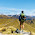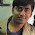## Binary Tree Maximum Path Sum

Given a binary tree, find the maximum path sum.
The path may start and end at any node in the tree.
For example:
Given the below binary tree,
       1
/ \
2   3

Return 6.

### Analysis (updated 2016.4):

At first glance, this problem is so 'unstable' that any node and any path could be the result path. Besides, there might be negative values in the tree nodes, which looks more annoying.

The example tree is so simple, let's make it a little more complex:
1
/    \
2     -3
/  \    /   \
4    5  6   -7
/  \    \
6   -1  -3

Then consider the problem in this way:
For any node in the tree, what makes a path go through it?
(1)  A path where the "top node" is the current node. E.g.,  node:2,   path: 6->4->2->5->-3
(2)  A path where the "top node" is the parent of current node. E.g., node:2, path: -3->5->2->1->-3->6

Now we consider the max path sum of node a
Denote max_single(a) as the path sum of case (2) above.
Denote max_top(a) as the path sum of case (1) above.

max_single(a) = MAX{ max_single(a.left)+a.val, max_single(a.right)+a.val, a.val}

max_top(a) = MAX{max_single(a), max_single(a.left)+max_single(a.right)+a.val, a.val}

(Note: a.val is the maximum if left and right sum are all negative)

To get the max path sum for every node a:
res = max(res, max_top(a))
(Don't forget to initialize "res" to INT_MIN)

For the whole algorithm, a simple recursion will work well and pass all the test cases.

### Code(C++):

/**
* Definition for a binary tree node.
* struct TreeNode {
*     int val;
*     TreeNode *left;
*     TreeNode *right;
*     TreeNode(int x) : val(x), left(NULL), right(NULL) {}
* };
*/
class Solution {
public:
int mxsum(TreeNode* root, int &res){
if (!root){
return 0;
}else{
int mx_l = mxsum(root->left, res);
int mx_r = mxsum(root->right, res);

int mx = max(max(mx_l+root->val, mx_r+root->val), root->val);
res = max(res, max(mx, mx_r+root->val+mx_l));
return mx;
}
}
int maxPathSum(TreeNode* root) {
int res = INT_MIN;
int mx = mxsum(root, res);
return res;
}
};


### Code(Python):

# Definition for a  binary tree node
# class TreeNode:
#     def __init__(self, x):
#         self.val = x
#         self.left = None
#         self.right = None

class Solution:
# @param root, a tree node
# @return an integer
res = None
def findmax(self, root):
if root == None:
return 0
else:
max_l = self.findmax(root.left)
max_r = self.findmax(root.right)
max_s = max(max(max_l, max_r) + root.val, root.val)
max_top = max(max(max_s, max_l+max_r+root.val), root.val)
if self.res == None:
self.res = max_top
else:
self.res = max(self.res, max_top)
return max_s

def maxPathSum(self, root):
self.findmax(root)
return self.res


1.good solutions!

2.Nice and neat!!

3.How to solve this problem using Dynamic programming ?

4.Could anyone tell me here in the complex tree above, what is the max sum?